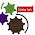## Saturday, 10 December 2016

### Sum of N No. of NATURAL Number - C Programming Examples

We have started C Programming coding with Example Series, We have published in previous post about Fibonacci , Arithmetic , Geometric And Harmonic Series.Now we are going to Explore new segment about Special Series and Sequences.

We have chosen to start with Natural Numbers and in this post you will find some basic idea and some useful concepts about Natural Numbers .In this post we are writing a C program for Finding SUM of N Natural Numbers in TURBO C/C++ Application Software / Compiler .You can download C Codes below.Please let us know your feedback.

SUM OF N NATURAL NUMBERS Video Tutorial(See C- Codes Below ):

Natural Numbers Explained:-
In mathematics, the natural numbers are those used for counting (as in "there are six coins on the table") and ordering (as in "this is the third largest city in the country"). In common language, words used for counting are "cardinal numbers" and words used for ordering are "ordinal numbers".

The sum of the first n natural numbers, Sn, is:Some authors and ISO 31-11 begin the natural numbers with 0, corresponding to the non-negative integers 0, 1, 2, 3, …, whereas others start with 1, corresponding to the positive integers 1, 2, 3, …
Texts that exclude zero from the natural numbers sometimes refer to the natural numbers together with zero as the whole numbers, but in other writings, that term is used instead for the integers (including negative integers).(REF)

Write A Program In C To Find out the Sum Of Series 1 + 2 + …. + n. Sum of Natural numbers to Nth Term:C Codes are written and tested in TuboC/C++ IDE as you can see in Video:

#include
int main()
{
int n,i; //Integer Initialized
int sum=0; //Variable sum initialized to Zero

printf("Enter the n i.e. max values of series: "); // Asks the user to enter the no. on terms
scanf("%d",&n); //Take Input from User

sum = (n * (n + 1)) / 2;  //SUM of Series is calculated

printf("Sum of the series: ");   // SUM of The Series(as TEXT) is displayed on Screen

for(i =1;i <= n;i++)
{
if (i!=n)
printf("%d + ",i);     //Individual Numbers are shown
else
printf("%d = %d ",i,sum);    // Sum of Series is Displayed after Equal Sign
}
delay(5000);
return 0;

}

1.What you're saying is completely true. I know that everybody must say the same thing, but I just think that you put it in a way that everyone can understand. I'm sure you'll reach so many people with what you've got to say.

1.Thanks For your encouraging words.We would like you to post in our guest section if you guys are intrested.

2.2.3.